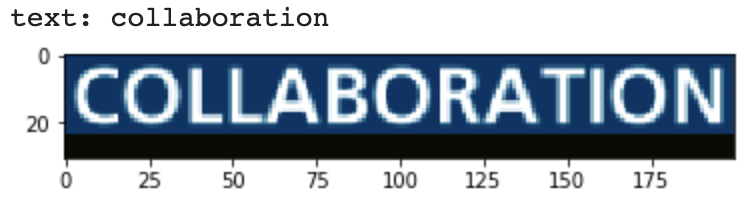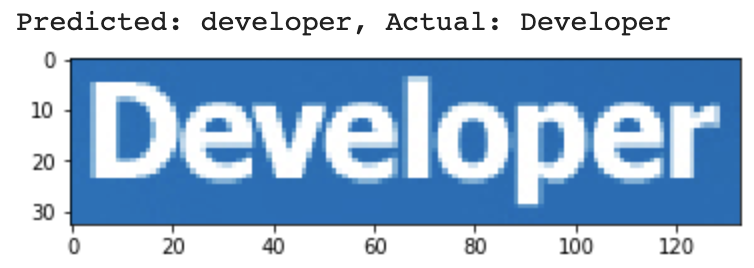# Fine-tuning the recognizer¶

This example shows how to fine-tune the recognizer using an existing dataset. In this case, we will use the “Born Digital” dataset from https://rrc.cvc.uab.es/?ch=1&com=downloads

First, we download our dataset. Below we get both the training and test datasets, but we only use the training dataset. The training dataset consists of a single folder containing images, each of which has a single word in it.

An interactive version of this example on Google Colab is provided here.

```import random
import string
import math
import itertools
import os

import numpy as np
import imgaug
import matplotlib.pyplot as plt
import tensorflow as tf
import sklearn.model_selection

import keras_ocr

assert tf.config.list_physical_devices('GPU'), 'No GPU is available.'

train_labels = keras_ocr.datasets.get_born_digital_recognizer_dataset(
split='train',
cache_dir='.'
)
test_labels = keras_ocr.datasets.get_born_digital_recognizer_dataset(
split='test',
cache_dir='.'
)
train_labels = [(filepath, box, word.lower()) for filepath, box, word in train_labels]
test_labels = [(filepath, box, word.lower()) for filepath, box, word in test_labels]
```

We next build our recognizer, using the default options to get a pretrained model.

```recognizer = keras_ocr.recognition.Recognizer()
recognizer.compile()
```

We need to convert our dataset into the format that `keras-ocr` requires. To do that, we have the following, which includes support for an augmenter to generate synthetically altered samples. Note that this code is set up to skip any characters that are not in the recognizer alphabet and that all labels are first converted to lowercase.

```batch_size = 8
augmenter = imgaug.augmenters.Sequential([
imgaug.augmenters.GammaContrast(gamma=(0.25, 3.0)),
])

train_labels, validation_labels = sklearn.model_selection.train_test_split(train_labels, test_size=0.2, random_state=42)
(training_image_gen, training_steps), (validation_image_gen, validation_steps) = [
(
keras_ocr.datasets.get_recognizer_image_generator(
labels=labels,
height=recognizer.model.input_shape,
width=recognizer.model.input_shape,
alphabet=recognizer.alphabet,
augmenter=augmenter
),
len(labels) // batch_size
) for labels, augmenter in [(train_labels, augmenter), (validation_labels, None)]
]
training_gen, validation_gen = [
recognizer.get_batch_generator(
image_generator=image_generator,
batch_size=batch_size
)
for image_generator in [training_image_gen, validation_image_gen]
]
```

As a sanity check, we show one of the samples.

```image, text = next(training_image_gen)
print('text:', text)
plt.imshow(image)
```Now we can run training.

```callbacks = [
tf.keras.callbacks.EarlyStopping(monitor='val_loss', min_delta=0, patience=10, restore_best_weights=False),
tf.keras.callbacks.ModelCheckpoint('recognizer_borndigital.h5', monitor='val_loss', save_best_only=True),
tf.keras.callbacks.CSVLogger('recognizer_borndigital.csv')
]
recognizer.training_model.fit_generator(
generator=training_gen,
steps_per_epoch=training_steps,
validation_steps=validation_steps,
validation_data=validation_gen,
callbacks=callbacks,
epochs=1000,
)
```

Finally, run inference on a test sample.

```image_filepath, _, actual = test_labels
predicted = recognizer.recognize(image_filepath)
print(f'Predicted: {predicted}, Actual: {actual}')```recognizer.model.load_weights('recognizer_borndigital.h5')